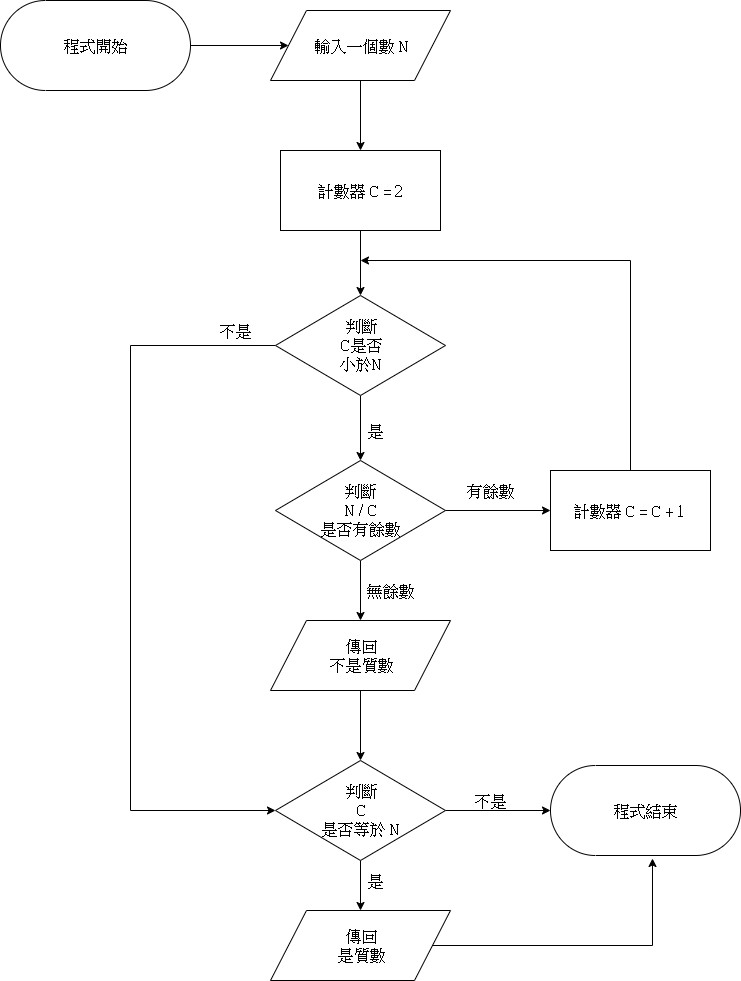# 質數判斷演算法

### 演算法

1. 輸入一個數 N
2. 設定 計數器 C = 2
3. 判斷 C 是否小於 N:
小於 N, 進行下一步
不小於N, 跳到步驟 7
4. 判斷 N 除以 C 是否有餘數:
無餘數表示整除, 傳回 "不是質數", 跳到步驟 7
有餘數表示不能整除, 進行下一步
5. 計數器 C = C + 1
6. 跳到步驟 3
7. 判斷 C 是否等於 N:
是, 傳回 "是質數"
不是, 進行下一步
8. 程式結束

### 流程圖### Python程式 while 寫法

#程式開始
n = int(input('輸入一個正整數:'))
c = 2

while c < n:
if n % c == 0:
print('不是質數')
break
c += 1

if c == n:
print('是質數')
#程式結束


### Python程式(for迴圈寫法)

#程式開始
n = int(input('輸入一個正整數:'))

for c in range(2,n):
if n % c == 0:
print('不是質數')
break
elif c == n-1:
print('是質數')

if n == 2:
print('是質數')
#程式結束


### Python程式(function寫法)

#程式開始
def is_prime(number):
for i in range(2,number):
if number % i == 0:
return False
return True

n = int(input('輸入一個正整數:'))

if is_prime(n):
print('是質數')
else:
print('不是質數')
#程式結束

#Python #質數 #演算法